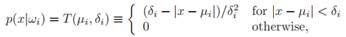# Generalize the minimax decision rule in order to classify patterns from three categories having triangle densities as follows: where δi > 0 is the half-width of the distribution (i = 1, 2, 3). Assume for convenience that µ1 <>2 <>3, and make some minor simplifying assumptions about the δi’s as needed, to answer the following: (a) In terms of the priors P(ωi), means and half-widths, find the optimal decision points x∗ 1 and x∗ 2 under a zero-one (categorization) loss. (b) Generalize the minimax decision rule to two decision points, x∗ 1 and x∗ 2 for such triangular distributions. (c) Let {µi, δi} = {0, 1}, {.5, .5}, and {1, 1}. Find the minimax decision rule (i.e., x∗ 1 and x∗ 2) for this case. (d) What is the minimax risk?

Question
2 views

Generalize the minimax decision rule in order to classify patterns from three categories having triangle densities as follows:where δi > 0 is the half-width of the distribution (i = 1, 2, 3). Assume for convenience that µ1 <>2 <>3, and make some minor simplifying assumptions about the δi’s as needed, to answer the following:

(a) In terms of the priors P(ωi), means and half-widths, find the optimal decision points x∗ 1 and x∗ 2 under a zero-one (categorization) loss.

(b) Generalize the minimax decision rule to two decision points, x∗ 1 and x∗ 2 for such triangular distributions.

(c) Let {µi, δi} = {0, 1}, {.5, .5}, and {1, 1}. Find the minimax decision rule (i.e., x∗ 1 and x∗ 2) for this case. (d) What is the minimax risk?

### This question hasn't been answered yet.